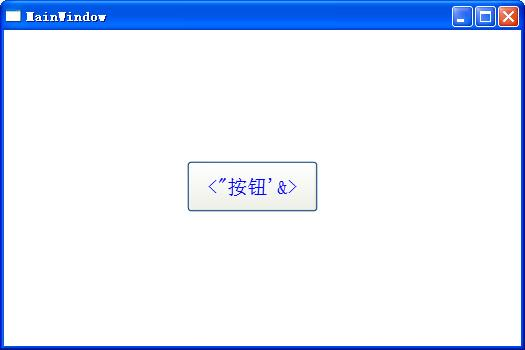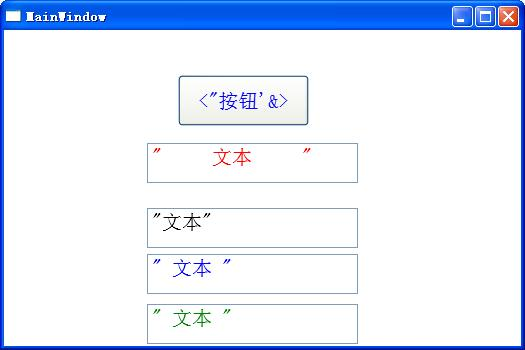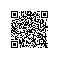# XAML中的特殊符号几空白字符处理

## 介绍

XAML标记语言是基于xml的，所以很多xml中的特殊符号在XAML也是需要处理的。

## 详细

（取自msdn）

Entity

&(“and”符)

&amp;

>（大于号字符）

&gt;

<（小于号字符）

&lt;

"（直双引号）

&quot;

'（直单引号）

&apos;

（数字字符映射）

&#[integer]; 或者 &#x[hex];

XAML 支持将数字字符映射到处于活动状态的编码。

（不间断空格）

&#160;（采用 UTF-8 编码）

## 处理

1 <Window x:Class="WpfApplication6.MainWindow"
2         xmlns="http://schemas.microsoft.com/winfx/2006/xaml/presentation"
3         xmlns:x="http://schemas.microsoft.com/winfx/2006/xaml"
4         Title="MainWindow" Height="350" Width="525">
5     <Grid>
6         <Button Margin="183,131,203,134" FontSize="20" Foreground="Blue">&lt;&quot;按钮&apos;&amp;&gt;</Button>
7     </Grid>
8 </Window>1 <Window x:Class="WpfApplication6.MainWindow"
2         xmlns="http://schemas.microsoft.com/winfx/2006/xaml/presentation"
3         xmlns:x="http://schemas.microsoft.com/winfx/2006/xaml"
4         Title="MainWindow" Height="350" Width="525">
5     <Grid>
6         <Button Margin="174,45,212,220" FontSize="20" Foreground="Blue">&lt;&quot;按钮&apos;&amp;&gt;</Button>
7         <TextBox Margin="143,110,163,161" Height="40" FontSize="20" Foreground="Red" xml:space="preserve">"     文本     "</TextBox>
8         <TextBox Margin="143,175,163,96" Height="40" FontSize="20" Foreground="Black">"文本"</TextBox>
9         <TextBox Margin="143,221,163,50" Height="40" FontSize="20" Foreground="Blue">" 文本 "</TextBox>
10         <TextBox Margin="143,271,163,0" Height="40" FontSize="20" Foreground="Green">"      文本      "</TextBox>
11     </Grid>
12 </Window>## Demo下载使用钉钉扫一扫加入圈子
+ 订阅